• 定义含参量函数 matlab
2021-04-19 00:51:33

%主文件：

clc

clear

a=input('输入参数a=')

x=linspace(0,100,500);

y=chengfa(a,x);

plot(x,y)

%%%%%%%%%%%%%%%%%%%%%

%函数文件：

function y=chengfa(a,x)

y=a*x;

再问： 能否不输入a，使得输出结果里直接包含a参数？

再答： 如果想不给a赋值，就要定义符号a. clc clear %a=input('输入参数a=') x=linspace(0,100,500); y=chengfa(x) %%%%%%%%%%%%%%%%% function y=chengfa(x) syms a y=a*x; %%%%%%%%%%%%%%%%%%%% 顺便说一下，你挺好学的。

再问： 最后一个问题，定义符号a的话是否就无法画图了，或者说，带有符号的多项式难以求极值了吧？

再答： a没有具体数值一定不能绘图。 通过绘图法求极值是比较粗略的方法。 即使能求出带符号a函数的极值表达式，也一定要赋值才能知道最后结果。 例如：syms a x >> t=solve('a*x^2-x+1=0') t = [ 1/2/a*(1+(1-4*a)^(1/2))] [ 1/2/a*(1-(1-4*a)^(1/2))] 最后还是要带入a的值才行。 顺便再说一句，要坚持你爱思考，爱提问题的学习态度。有什么问题尽管提问。现在认真学习的同学真的不多了。

更多相关内容
• RA=ROUTH(R,EPSILON) 返回多项式的符号劳斯数组 RA。 考虑以下特殊情况： 1) 如果一行的第一个元素变为零或者2) 如果遇到一行全是零。 >>syms ep >>a=routh([1 1 2 2 3 5],ep) 上面给出的情况是在第一列中遇到零。
• 其余的程序语句和C++大同小异，当然matlab也可以和C++一样自己定义函数定义函数语句为: function输出形参=函数名(输入形参) 例: 其中c为输出形参，counum为函数名，a,b为输入形参，函数功能为后续语句。当你需要...
• INLINEDEF 提供了在脚本中定义函数或类的外观（减去语法突出显示），尽管包含函数或类定义的单独 M 文件实际上是自动生成的并保存在 Matlab 路径上 - 并且没有更具体的范围。 与直接创建 M 文件相比，使用 INLINEDEF...
• matlab 定义函数,matlab定义函数并调用,Matlab自定义函数详解很久以前写的一篇Matlab自定义函数访问量很大，可惜没有点赞的，我感觉是我没讲清楚，这里又写了一篇笔记Matlab函数函数是组织好的，可重复使用的，用来...

matlab 定义函数,matlab定义函数并调用,Matlab自定义函数详解

很久以前写的一篇Matlab自定义函数访问量很大，可惜没有点赞的，我感觉是我没讲清楚，这里又写了一篇笔记

Matlab函数

函数是组织好的，可重复使用的，用来实现单一，或相关联功能的代码段。

函数能提高应用的模块性，和代码的重复利用率。你已经知道Matlab提供了许多内建函数，比如disp()。但你也可以自己创建函数，这被叫做用户自定义函数

disp('hello world')

hello world

总的来说，自定义函数分为两步：即定义函数和调用函数。

定义一个函数

你可以定义一个由自己想要功能的函数，以下是简单的规则：

函数代码块以 function关键词开头，后接输出变量和函数标识符名称和圆括号 ()。

任何传入参数和自变量必须放在圆括号中间，圆括号之间可以用于定义参数。

函数的第一行语句可以选择性地使用文档字符串—用于存放函数说明。

函数内容以end结束。

函数需要定义在脚本文件(*.m)中运行，不可以在命令窗口中临时创建。

Matlab定义函数需要使用function关键字，一般格式如下：

function 输出变量 = 函数名(输入变量)

这里的输入变量和输出变量都可以根据实际情况缺省。

创建一个函数，用来输出“hello world!” ，步骤如下：

新建一个脚本，命名为eg1.m

test();

function test()

disp('hello world!')

点击顶部菜单的运行按钮，即可在命令窗口输出：

eg1

hello world!

更复杂一点的应用，我们让函数带上输入变量和输出变量：

s = calc_area(4,5);

function s = calc_area(w,h)

s = w*h;

disp(['area=',num2str(s)]);

以上实例输出：

eg2

area=20

输入变量缺省

有时候，输入变量的个数不确定，但是又想将不同个数情况统一到一个函数里，即输入变量有缺省时，可以利用nargin来判断，然后给出对应的输出。例如：

out(1)

out(1,2)

out(1,2,3)

function out(a,b,c)

if nargin == 1

disp(a)

elseif nargin == 2

disp([a,b])

elseif nargin == 3

disp([a,b,c])

上面的实例将会得到：

eg5

1 2 3

可以看到，当输入不同输入变量个数时，会找到对应个数下的执行代码，返回相应的内容。

当然这种做法也存在弊端，当变量个数太多时，要写很多个判断；这种情况下可以尝试inputParser,这里我就不多解释了，有兴趣可以了解下。

函数基本结构完成后，进行调用时，存在两种方法：

直接在函数所在脚本文件内调用(上面的例子就是这种情况)；

函数定义与调用分开，各自存为一个脚本，运行调用脚本。

法一：脚本内调用函数如上图所示，调用函数在前，定义函数在后，点运行即可得到输出。

法二：函数脚本独立，另外写代码调用如上图所示，函数脚本和调用函数的脚本分开了，保存在同一路径下。

在第二种方法下，也可以在命令窗口直接调用所写的函数：这种调用与上面本质上是一样的，都是首先找到以函数名命名的脚本文件，然后再执行函数内部代码块，返回结果。在这里必须注意函数脚本名字要与函数名一致。

事实上，为了让编程变得系统化，模块化，我们不建议你采用第一种方法去写代码，因为这样不利于分析和维护代码。

而采用后面一种方法时，我们可以定义很多个函数，然后在其他脚本里调用它们，这样形成了一种模块化的关系。我们在分析代码时，注意力可以集中到主程序上，思路将更加清晰。

输出值缺省调用

在matlab的函数中，可以选择性地获取所返回的变量列表值，下面给出实例：

default = out()

[a,~,~]= out()

[~,b,~] = out()

[~,~,c] = out()

function [a,b,c] = out()

a = 1;

b = 2;

c = 3;

上述实例输出结果：

eg4

default =

展开全文• 当我们在使用Matlab的时候，因需求要进行定义函数，有些朋友还不熟悉操作方法?下面一起去看看Matlab定义函数的详细操作教程，相信你会喜欢的。Matlab定义函数的详细操作教程方法一：通过inline函数定义函数打开...

当我们在使用Matlab的时候，因需求要进行定义函数，有些朋友还不熟悉操作方法?下面一起去看看Matlab定义函数的详细操作教程，相信你会喜欢的。

Matlab定义函数的详细操作教程方法一：通过inline函数定义函数

打开matlab，在命令行窗口中输入“f=inline('5*x+1','x')”，按回车键创建f(x)=5*x+1内联函数，如图所示：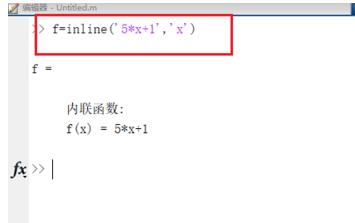输入“x=3;f(x)”，按回车键，可以得到f(x)等于16，如图所示：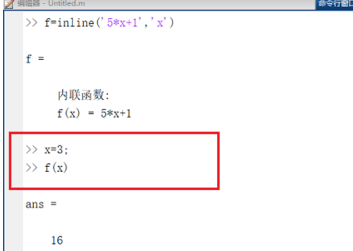方法二：匿名函数定义

在命令行窗口中输入“f=@(x)5*x+3”，按回车键定义函数f=@(x)5*x+3，如图所示：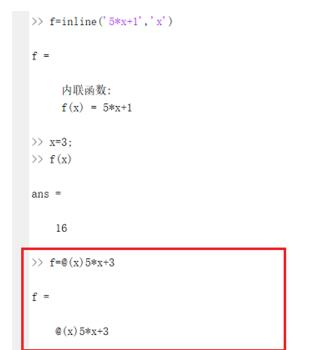输入“x=2;f(x)”，按回车键，得到结果13，如图所示：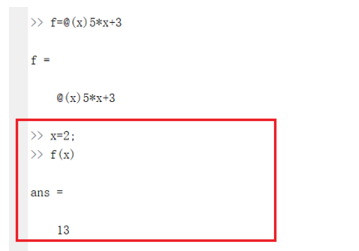方法三：创建m函数

在matlab中点击新建，选择函数，如图所示：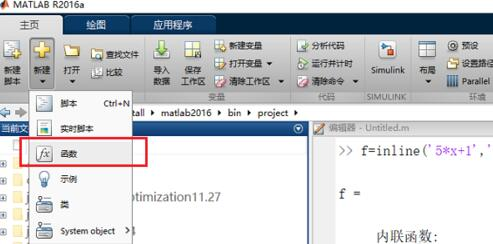可以看到已创建了m函数，可在脚本文件中调用函数，如图所示：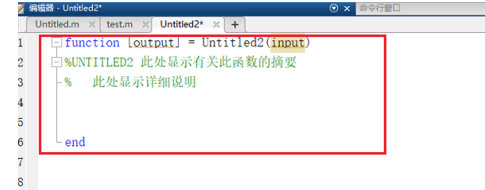Matlab相关攻略推荐:

各位小伙伴们，看完上面的精彩内容，都清楚Matlab定义函数的详细操作了吧!

展开全文• 有不少用户在使用电脑的过程中可能会碰到matlab定义函数的情况。大部分用户电脑技术都是菜鸟水平，面对matlab定义函数的问题，无法自行解决。不少用户都来咨询小编matlab定义函数要怎么处理？其实可以根据以下步骤1...

有不少用户在使用电脑的过程中可能会碰到matlab定义函数的情况。大部分用户电脑技术都是菜鸟水平，面对matlab定义函数的问题，无法自行解决。不少用户都来咨询小编matlab定义函数要怎么处理？其实可以根据以下步骤

1：打开编译器,在命令行输入edit打开

2：输入Function代表此文件是函数文件

就可以搞定了。下面就和小编一起看看解决matlab定义函数问题详细的操作步骤：

1：打开编译器,在命令行输入edit打开2：输入Function代表此文件是函数文件3：如果有返回值在等号的左边输入,多个返回值用中括号4：等号右面是函数名加括号,括号内是参数5：使用%注释,注释的第一行是说明函数的功能6：传入两个点的坐标,之后计算两点的距离,传给返回值y7：保存文件,保存的时候文件名和函数名保持一致8：因为函数不能直接执行,需要传参,定义两个坐标值x1 =2,x2 =4,y1 = 4,y2 = 49：定义计算结果变量result = dist2(x1,y1,x2,y2)，得出结果10：这就是函数编程的基础部分,喜欢就点个赞吧

展开全文• 使用“Abramowitz 和 Stegun：数学函数手册”中的级数定义计算 struve 函数。 它似乎相当准确，也不是那么慢，但它肯定没有优化。 请参阅（修改后的 struve 函数的 struvem.m）
• 下面是几种窗函数归一化DTFT幅度的MATLAB程序:
• 自定义函数，tstem(hn,y)，可以用来作离散序列柄状图。
• 下面就总结一下如何在MATLAB定义函数。同时matlab支持多输入多输出，函数的返回值可以为一个向量，矩阵，或者多个元素。为了让函数可以调用，需要对函数进行命名。matlab支持多输入多输出，函...
•开发语言 矩阵
• 请教各位怎样用matlab定义一个分段函数 ...语句定义不同的分支推荐方法：使用逻辑表达式将不同分支写成一个完整的函数表达式下面进行举例说明：在matlab定义如下分段函数1、常规方法：123456789functiony=fun(x...
• 使用matlab做任意长度的DFT，绘制时域频域图像，对比。更改N为时域信号长度，L为做DFT点数。N，则补零。N,L可更改参数。
• 1 误差函数定义为 它的性质如下 2 互补误差函数定义为 它具有如下性质 3...Q 函数写成 erfc函数matlab 中有此函数的形式 另一种就是通过上述两者的关系定义一个函数文件即Q函数文件这样就可以直接使用Q函数了 a=2; p_er
• 虚数误差函数，如 Mathematica 中定义的 erfi(z)==erf(iz)/i（z 可能是复数），使用 matlab 中的不完全伽马函数： gammainc
• PAGE / NUMPAGES Matlab 自定义函数 ?1函数文件+调用命令文件需单独定义一个自定义函数的M文件; ?2函数文件+子函数定义一个具有多个自定义函数的M文件 ?3Inline:无需M文件直接定义 ?4Syms+subs?无需M文件,直接定义 ?...
• 分段函数定义分段函数;对于自变量x的不同的取值...求matlab中带符号二进制转化为十进制函数?txt中数据转化为二进制.怎么办?其次,本次作业要求我们图片进行哈夫曼编码使用dec2bin()函数b=double(b);[MN]=size(b);fo...
• matlab开发-内联定义函数inscripts。在脚本中定义函数和/或类。数据分析和机器学习
• Matlab函数、子函数的定义方法除了最常使用的m文件函数，matlab还可以通过内联函数和匿名函数自定义函数。1. m文件函数以function关键字...2. 子函数matlab可以在m文件函数中定义一个函数和多个子函数，但是需要注...
• Matlab函数定义方法Matlab自定义函数的六种方法n1、函数文件+调用函数(命令)文件：需单独定义一个自定义函数的M文件；n2、函数文件+子函数定义一个具有多个自定义函数的M文件；n3、Inline:无需M文件，直接定义；...
• matlab符号函数定义: 关于函数符号的介绍，缺失：matlab符号定义3698/9约翰．伯努利於1694年首次提出函数(function)概念，并以字母 n 表示变量 z 的一个函数；至 1697年，他又以大写字母 X 及相应之希腊字母 ξ表示...
• 点击查看MATLAB中此上下文中不允许出现函数定义，急求~具体信息答：从你讲的问题，虽然没讲太清楚，但我推测，你可能写了个脚本程序文件，其中定义了子函数，在运行时就弹出了这个提示。一般情况下，在函数文件中...
• ## Matlab定义函数的几种方法

万次阅读 多人点赞 2017-09-07 15:20:13
1、函数文件+调用命令文件：需单独定义一个自定义函数的M文件 例：自定义求和函数 需要自定义一个 my_sum.m 的文件来存放函数 function[s]=my_sum(n) s=0; for i=1:n s=s+i; end注意：函数名（my_sum）必须...
• 使用matlab语言，编写PSO算法以及两种适应度函数Rastrigin和Griewank
• Matlab函数定义和调用 Matlab 自定义函数的五种方式 命令文件/函数文件+ 函数文件 多个 M 文件 自定义函数的五种方式 函数文件 + 子函数 一个 M 文件 inline 无需 M 文件 符号表达式 + subs 方式 无需 M 文件 字符串...
• ## matlab如何在文中定义函数

万次阅读 多人点赞 2017-01-04 14:12:54
matlab如何在文中定义函数 通常我们多是将函数单独在编写在单个的m文件中，再于主程序中调用。但是，有时候觉得程序太小，直接在程序末尾编写函数，又会收到“此上下文中不允许函数定义”的错误提示。那么，matlab...脚本
• 1、函数文件的基本结构 function 输出形参表=函数名（输入形参表) 注释说明部分 函数体语句 当有多个形参时，形参之间用逗号分隔，组成形参表。当输出形参多于一个时，应该用方括号括起来，构成一个输出矩阵。 函数...
• function [dev] = get_deviations(ws) #这个是定义函数 x = length(ws) ; sum = 0 ; for i = 1:x sum = sum + ws(i) ; end ave = sum/x ; for i = 1:x dev(i) = ws(i) - ave ; end for i = 1:x #使用定义函数 ......

# 怎么定义函数matlabmatlab 订阅